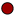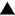Algebra 2 section of MathBitsNotebook.comPolynomialsTopic Standards:NGMSCCSS

* Check out the Refresher section at the bottom of this page if you need to refresh old skills.Factoring and Perfect Cubes  (a3 + b3  and  a3 - b3)Polynomial Remainder Theorem (and Factor Theorem)++

*
*

Polynomial Graphs (zeros, multiplicity, end behavior, graph behavior near roots)See Refresher Section below for more graphing information.

*+

 PRACTICE: • Practice with Factoring by Grouping• Practice with Polynomial Division• Practice with Polynomial Equations (multiple choice)• Practice with More Polynomial Equations (solutions shown)• Practice with Polynomial Identities+ • Systems: Linear - Quadratic Practice (found under Quadratic section) • Practice with Systems 3x3 • Practice Graphing Polynomial Functions• Practice with Binomial Theorem+ • Practice Working with PolynomialsREFRESHERS: (while these topics are mostly "review", you may also find new information here) * • Polynomials (definition, degree, leading term, constant, standard form, closure)• Polynomials as a System• Polynomials - Add, Subtract, Multiply • Polynomials - Divide (only monomial or factorable for binomial) • To refresh basic skills with Factoring Polynomials, see Factoring in Algebra 1. • General Features of Function Graphs (intercepts, positive/negative, increase/decrease)* • More Features of Function Graphs (continuous, max/min, symmetry, end behavior)• Transformations of Functions